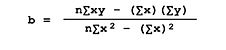top of page#### Statistical Correlation/Regresson

An explanation that contains predictions should at a minimum not only rule out most alternative theories, it should establish its theory as more credible than its negation.  [Also, within]…models that generate data, the expectations are that the generated data will correspond with the real data corpus.

[Zubrow 1973:245-6]

The problem of formulating a system of proportion may now be dealt with from a perspective of ordered sets. One statistical technique designed to show relationships between proportional variables is the linear regression equation. This statistical method attempts to predict the exact value of one component as it relates to another by using a straight-line relationship between the two variables [Blalock 1960].  The coordinates for two sets of values are plotted on a graph and follows the path through the points that represent the average deviated value. This path “…minimizes the sum of the squared deviations of points from the line, that is, the least-square best fit” [Yates 1974:68].  Thus the sum of the squares of the distances of vertical lines from each data point to the least-square line will result in a number of less than a “…comparable sum of squares from any other straight line” [Blalock 1960:373].

“Once this ‘best fit’ line has been determined, it is characterized by parameter 'a' the intercept of the line with the 'Y' axis, and parameter 'b' the regression coefficient, which indicates the slope of the line. In the simplest terms, the calculated line shows that for every unit increase in one set of coordinates, a corresponding unit increase can be precisely determined in the value of the other set of coordinates. [Yates 1974:69]

The formula for calculating proportional means, which determines clustering along a straight line for two sets of variables, is Y=a+bx, where 'Y' is the independent variable; 'x' is the dependent variable; 'a' is the intercept with the 'Y' axis; and 'b' is the co-variation of 'x' and 'Y,' divided by the sum of the squares in 'x' and represents the slope of the line.

The formula for measuring the amount of change in 'y' with each unit change of 'x' is 'b' (regression):

[Blalock 1972:373]

The following is a list of the variables used for the statistical test:

Dependent Variable: the axial peristyle which defines the proportional rectangle (AXLL).

Independent Variables:

• width of temple using the sum of column interaxial measurements (AXLW).

• column base diameter (COLW)

• column height (COLH)

• triglyph & metope width (TRI-METW

The following is a list of the temples used for the sample where accurate values are available.

≠ It should be noted that some structures are so poorly preserved that only the stylobate and/or portions of the crepis are preserved. In these cases, the excavators have attempted to form their reconstructions based on the best available information. When this occurs, the actual interaxial measurements are only estimates.bottom of page